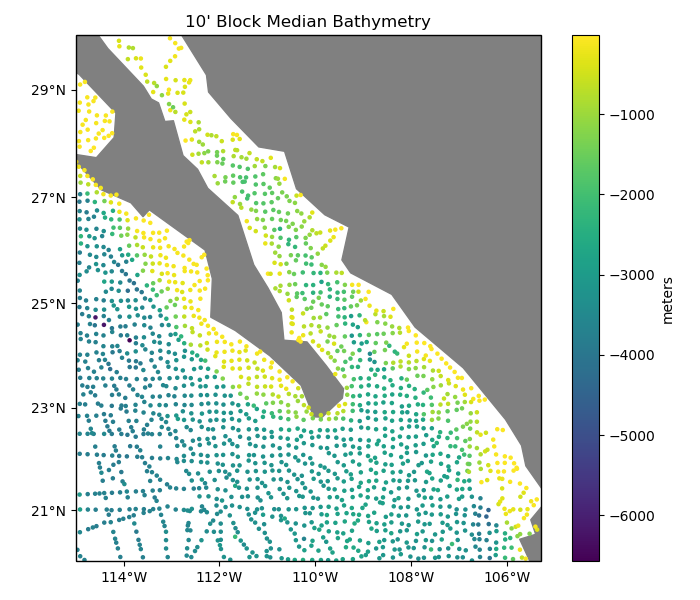# Blocked reduction operations¶

When gridding data that has been highly oversampled in a direction (shipborne and airborne data, for example), it is important to decimate the data before interpolation to avoid aliasing. Class verde.BlockReduce decimates data by applying a reduction operation (mean, median, mode, max, etc) to the data in blocks. For non-smooth data, like bathymetry, a blocked median filter is a good choice.Out:

Original data size: 82970
Decimated data size: 1801


import matplotlib.pyplot as plt
import cartopy.crs as ccrs
import numpy as np
import verde as vd

# We'll test this on the Baja California shipborne bathymetry data
data = vd.datasets.fetch_baja_bathymetry()

# Decimate the data using a blocked median with 10 arc-minute blocks
reducer = vd.BlockReduce(reduction=np.median, spacing=10 / 60)
coordinates, bathymetry = reducer.filter(
(data.longitude, data.latitude), data.bathymetry_m
)
lon, lat = coordinates

print("Original data size:", data.bathymetry_m.size)
print("Decimated data size:", bathymetry.size)

# Make a plot of the decimated data using Cartopy
plt.figure(figsize=(7, 6))
ax = plt.axes(projection=ccrs.Mercator())
ax.set_title("10' Block Median Bathymetry")
# Plot the bathymetry as colored circles.
plt.scatter(lon, lat, c=bathymetry, s=5, transform=ccrs.PlateCarree())
plt.colorbar().set_label("meters")
# Use a utility function to setup the tick labels and land feature
vd.datasets.setup_baja_bathymetry_map(ax)
plt.tight_layout()
plt.show()


Total running time of the script: ( 0 minutes 0.548 seconds)

Gallery generated by Sphinx-Gallery